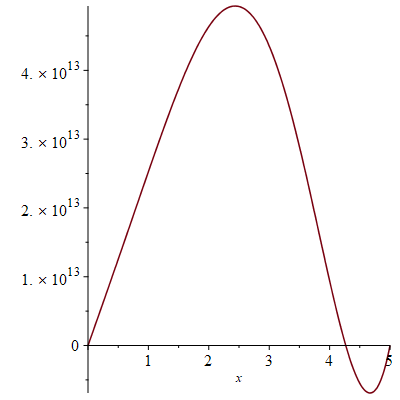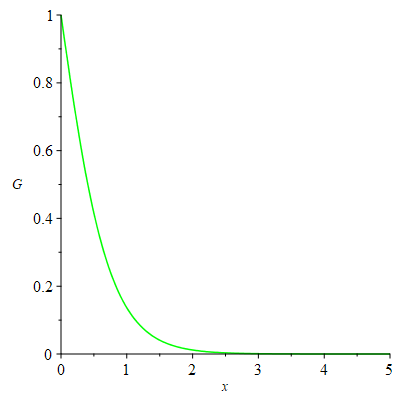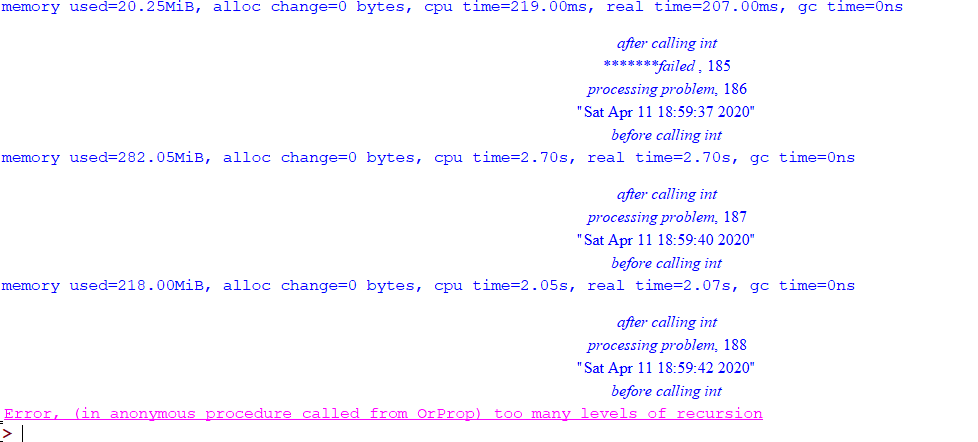## Maple 2018 recently become sluggish to start up ...

Maple 2018  has recently has  become sluggish to start up  -and very slow to respond to input. Can anyone suggest remedies?  I have plenty of space and CPU. Other apps seem to start fine.  Can any suggest a diagnosis and/or solution?

Melvin

## Converting Maple Tutor output to LaTeX...

Maple Tutors allow for copying of MathML but I need LaTeX of it. Any help please?

## How to plot Bloch sphere on maple?...

If we have an equation for the generalized Bloch sphere i.e.,

\partial_{t}(u^{2} + v^{2} + w^{2}) = 0,

where u, v and w are functions of x and t and the initial conditions u=v=0, w=-1. Then how to plot this equation on maple?

## round function...

Being an absolut newbie to Maple I find some of the functions a bit strange and difficult.

One of the things is e.g. rounding numbers.

In Pyton and other languages it is quite simple and straightforward when rounding a number.

round(number, digits)

If you have digits = 0 then you get an integer, otherwise you get a number with the number of digits chosen.

In Maple the round function is just for rounding to an integer.

To round to something else currently I don't really know what I would use. There is the evalf function, which one can use like evalf[digits](number), but that doesn't give you the value with digits. It gives you a number with significant digits, which means, also including the numbers before the comma delimiter.

Python
round(15.76543,2) = 15.77

Maple
evalf(15.76543) = 16

Wouldn't it be much easier to expand the functionality of the round function in Maple?

## Maple Companion App Trouble...

"Frame your math" does not work on a new iPhone XS; however, surprisingly, it work just fine on an old iPad (4th gen).

I am logged in. The app has access to the camera. The companion app does allow manual entry of equation to upload to the Maple cloud.

I expect it's something simple--like some sort of permissions setting, but I cannot find it . . .

BTW: The error is "The request timed out. Check your Internet connection."

## Vector issues and Excel .csv ArrayTools Append bug...

Noticed an issue with Vector and excel.  Not even really excel but I found it through that.

If I select a row in excel and do a copy paste into Maple it sometimes appears as if it's a list.  ie it shows up as [1,2,3,4]  other times it shows up as [ 1 2 3 4 ] signifying an array (for this example I've saved as the data under the variable a).  Running whattype(a) on the data shows they're both arrays.  Of course it's probably wise to instead load data through Excel into Maple by using the ExcelTools and Import option, however I didn't think there was no reason to use the copy paste option.  It does, to some extent, or maybe, well, it shows a limitation.

In the first instance when it shows up as a list, I thought it might be simple enough just to add other numbers in the list ie/ just tack on ,5,6,7,8 after the 4.  But after pressing enter we get the error

Error, invalid sequence

I don't understand why I couldn't just add to the copied data but maybe it's the formatting of the data that was pasted into maple?

With the original copied data, I can use with(plots):  listplot(a) no problem however, we want to add data.  ArrayTools will let us Append data to the vector

with(plots):
with(ArrayTools):
Append(a,5,6,7,8)

listplot(a) # will throw another error

Error, (in plots:-pointplot) points cannot be converted to floating-point values (this doesn't show in Maple 2019 but does come up in Maple 18)

For Maple 2019 it with responds with a returned array [ 1 2 3 4 5,6,7,8]

Running listplot on that Appended Array.  Maple only recognizes the next 1 added value.

So this means appending values using ArrayTools and Append only allows us to append one value at a time?  Is that a bug?  Are there better ways to append values to vectors?  The only solution for that is to convert to a list and use op to append more values.  But perhaps Maple should have understood the copied values from Excel should have been a list or listlist of values?

## please any body can check mistake in problem, my H...

Hompotopy perturbation method

restart; with(LinearAlgebra);
PDEtools[declare](f(x), prime = x);
PDEtools[declare](g(x), prime = x);
N := 3;
F := sum(p^i*f[i](x), i = 0 .. N);
G := sum(p^i*g[i](x), i = 0 .. N);
FEq := (1-p)*(diff(F, x$3))+p*(diff(F, x$3)-(2*(diff(F, x$1))*(diff(F, x$1))-(5/2*F)*(diff(F, x$2))+M*(diff(F, x$1))) . A);
GEq := (1-p)*(diff(G, x$2))+p*(diff(G, x$2)+(5/2*(1/(1+R)) . Pr)*F*(diff(G, x$1)))*K(L); M := 1; A := (((1-W)^2.5*(1-W))*&rho;f+W*&rho;t)/&rho;f; Pr := 6.2; K := ((1-W)*&rho;f*Cf+W*&rho;t*Cs)/&rho;f; L := .5; &rho;f := 998.3; &rho;t := 3970; W := .2; R := 1.0; Cs := 765; Cf := 4182; coeff(FEq, p, 0); coeff(GEq, p, 0); for i from 0 to N do Fequ[i] := coeff(FEq, p, i) = 0 end do; for i from 0 to N do Gequ[i] := coeff(GEq, p, i) = 0 end do; Fcond := f(0) = 0, (D(f))(0) = 1, (D(f))(5) = 0; for j to N do Fcond[j] := f[j](0) = 0, (D(f[j]))(0) = 0, (D(f[j]))(5) = 0 end do; Gcond := g(0) = 1, g(5) = 0; for j to N do Gcond[j] := g[j](0) = 0, g[j](5) = 0 end do; for i from 0 to N do dsolve({Fequ[i], Fcond[i]}, f[i](x)); f[i](x) := rhs(%) end do; for i from 0 to N do dsolve({Gcond[i], Gequ[i]}, g[i](x)); g[i](x) := rhs(%) end do; Fa := simplify(sum(f[n](x), n = 0 .. N)); dFa := diff(Fa, x); subs(x = 2.4, dFa); Ga := simplify(sum(g[n](x), n = 0 .. N)); dGa := diff(Ga, x); subs(x = 2.4, dGa); plot(Ga, x = 0 .. 5); plot(dFa, x = 0 .. 5);ND sove solution Eq1 := diff(F(x), x$3)-(2*(diff(F(x), x$1))*(diff(F(x), x$1))-(5/2*F(x))*(diff(F(x), x$2))+M*(diff(F(x), x$1))) . A = 0;
Eq2 := (diff(G(x), x$2)+(5/2*(1/(1+R)))*Pr*F(x)*(diff(G(x), x$1)))*K(L) = 0;
M := 1;
Pr := 6.2;
A := (((1-W)^2.5*(1-W))*&rho;f+W*&rho;t)/&rho;f;
K := ((1-W)*&rho;f*Cf+W*&rho;t*Cs)/&rho;f;
L := 1;
&rho;f := 998.3;
&rho;t := 3970;
W := .2;
R := 2.0;
Cs := 765;
Cf := 4182;

Cd1 := F(0) = 0, (D(F))(0) = 1, (D(F))(5) = 0;
dsys := {Cd1, Eq1};
dsol := dsolve(dsys, numeric, output = operator);
dsol(.1);
plots[odeplot](dsol, [x, diff(F(x), x\$1)], 0 .. 5, color = green);
Cd2 := G(0) = 1, G(5) = 0;
dsys := {Cd1, Cd2, Eq1, Eq2};
dsol := dsolve(dsys, numeric, output = operator);
plots[odeplot](dsol, [x, G(x)], 0 .. 5, color = green)## Can maple do motion planning ? Which version can d...

I find some book teach motion planning in topology

but do it need to formulate the equations for the environments such as a map with obstacle in 2d or 3D?  the environment is quite complex, how can these equations be formulated?

## "How do I import all or part of PDF files?"...

how do i Import part or all  PDF files into maple

I believe this was easier in older versions of maple or worked partially.

## Error, (in OrProp) too many levels of recursion. t...

This is very strange problem, and it seems to happen at random times.

From my worksheet, I call a function in a local fille maple_proc.mpl. This function ends up calling int() command with a timeout.

I Have the int() call wrapped by try...catch:.. end try in order to catch timeout and any other error.

Yet, when I run my worksheet, looping over hundreds of integrals, for each one, calling the function in maple_proc to integrate it, and after running for many many integrals, then sometimes, the worksheet terminates with error, at some iteration with error

Error, (in OrProp) too many levels of recursion |maple_proc.mpl:45|

So the try/catch I have in place are not catching the above error, wherever it is coming from.

line 44 in maple_proc.mpl, just does this (it is the line with cpu_time := .... below

try
print(before calling int);
cpu_time := timelimit(180,CodeTools[Usage](assign('result_of_int',int(lst[i,1],lst[i,2])),output='realtime')):
print(after calling int);
catch "time expired":
#...
catch:
#...
end try;


Again, this works for sometimes for hundreds of iterations, and then on some entry, it gives this error.

I do not use recursion myself at all. i.e. I have no place where the function calls itself. Worksheet does a loop, and in each interation calls this function in maple_proc.mpl.

Sometimes, when I run the worksheet again, the error do not show up again. I have print statements in the catch above, and these do not show up at all, which means this error is not cought.

What should I do to find why this happens? This did not happen in Maple 2019.2. Is there any other way to trap this error so my loop does not terminate like this and I have to restart it again each time?

I am using Physics 642 and Maple 2020 on windows 10.

Update

I was able to strip all the code and make a MWE. There is a zip file attached.

one worksheet, which calls a maple_proc() function now in the same worksheet.

This function reads a plain text file in same folder, which contains list of integrals. The function maple_proc() does a "read" which reads all problems into a variable called lst

Now it simply does a LOOP over all entries in lst calling int() on each integral (one integral per line) with a timeout. You see there is catch() there.

I get the erorrAt iteration number 188 each time.

I will attach a ZIP file now, which contains one folder which contains the worksheet and the input plain textfile. All what you need to do to reproduce this is open the worksheet and evaluate the call there. You might want to edit the hardcoded folder in the worksheet to your own folder where you unzipped the zip file.

currentdirName :="C:/MAPLE_BUG";
currentdir(currentdirName);


I hope someone will be able to repoduce this. It looks like a real bug in Maple 2020.

The problem is not in the integral 188 itself. Because if the loop starts at say 100 or 120, instead from 1, the integral now is processed OK and no error is generated.

MAPLE_BUG.zip

## pdsolve in toroidal coordinates?...

How I can pdsolve these equations in toroidal coordinates?

Initial conditions are arbitrary.

Thanks

Where.pdf

Weston1958

## Can old maple version saved .m files in window be ...

Can old maple version saved .m files in window be readable in maple 2015 Linux version?

## How to preserve the order when extracting the coef...

Hello (again)

I thought I won't need help with that type of question but I came across an example that says otherwise.  Here it is

vars:=[x,y,z];

model7 := [x*(-RootOf(64*_Z^3+80*_Z^2+1104*_Z+561)-5/4)+y*alpha[1, 2]-33/(32*RootOf(64*_Z^3+80*_Z^2+1104*_Z+561)), x*z*alpha[2, 6]+y*RootOf(64*_Z^3+80*_Z^2+1104*_Z+561), x^2*(17*RootOf(64*_Z^3+80*_Z^2+1104*_Z+561)+17)/(alpha[1, 2]*alpha[2, 6])-17*x*y/alpha[2, 6]+2*z*x-z-(163/32+RootOf(64*_Z^3+80*_Z^2+1104*_Z+561)^2+5*RootOf(64*_Z^3+80*_Z^2+1104*_Z+561)*(1/4))/(alpha[1, 2]*alpha[2, 6])]


then I issued the command

map(w->coeffs(w,vars),model7);


to get

[-33/(32*RootOf(64*_Z^3+80*_Z^2+1104*_Z+561)), -RootOf(64*_Z^3+80*_Z^2+1104*_Z+561)-5/4, alpha[1, 2], RootOf(64*_Z^3+80*_Z^2+1104*_Z+561), alpha[2, 6], -(163/32+RootOf(64*_Z^3+80*_Z^2+1104*_Z+561)^2+5*RootOf(64*_Z^3+80*_Z^2+1104*_Z+561)*(1/4))/(alpha[1, 2]*alpha[2, 6]), -1, (17*RootOf(64*_Z^3+80*_Z^2+1104*_Z+561)+17)/(alpha[1, 2]*alpha[2, 6]), -17/alpha[2, 6], 2]

clearly the order does not follow model7's.

I have also tried

[seq(coeffs(expand(model7[i]), indets(model7[i], suffixed({vars[]}))),i=1..nops(model7))];

Is there a solution to it?

Many thanks (again)

Ed

## How to learn Maple programming effectively especia...

How to learn Maple Programming effectively, whether Python will help?